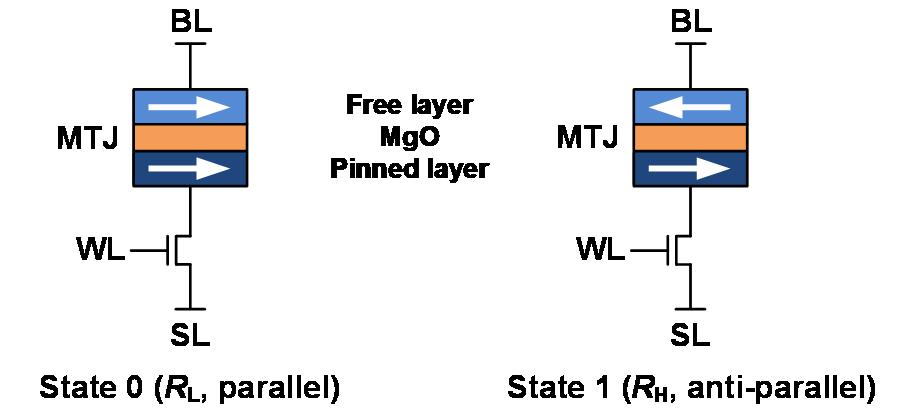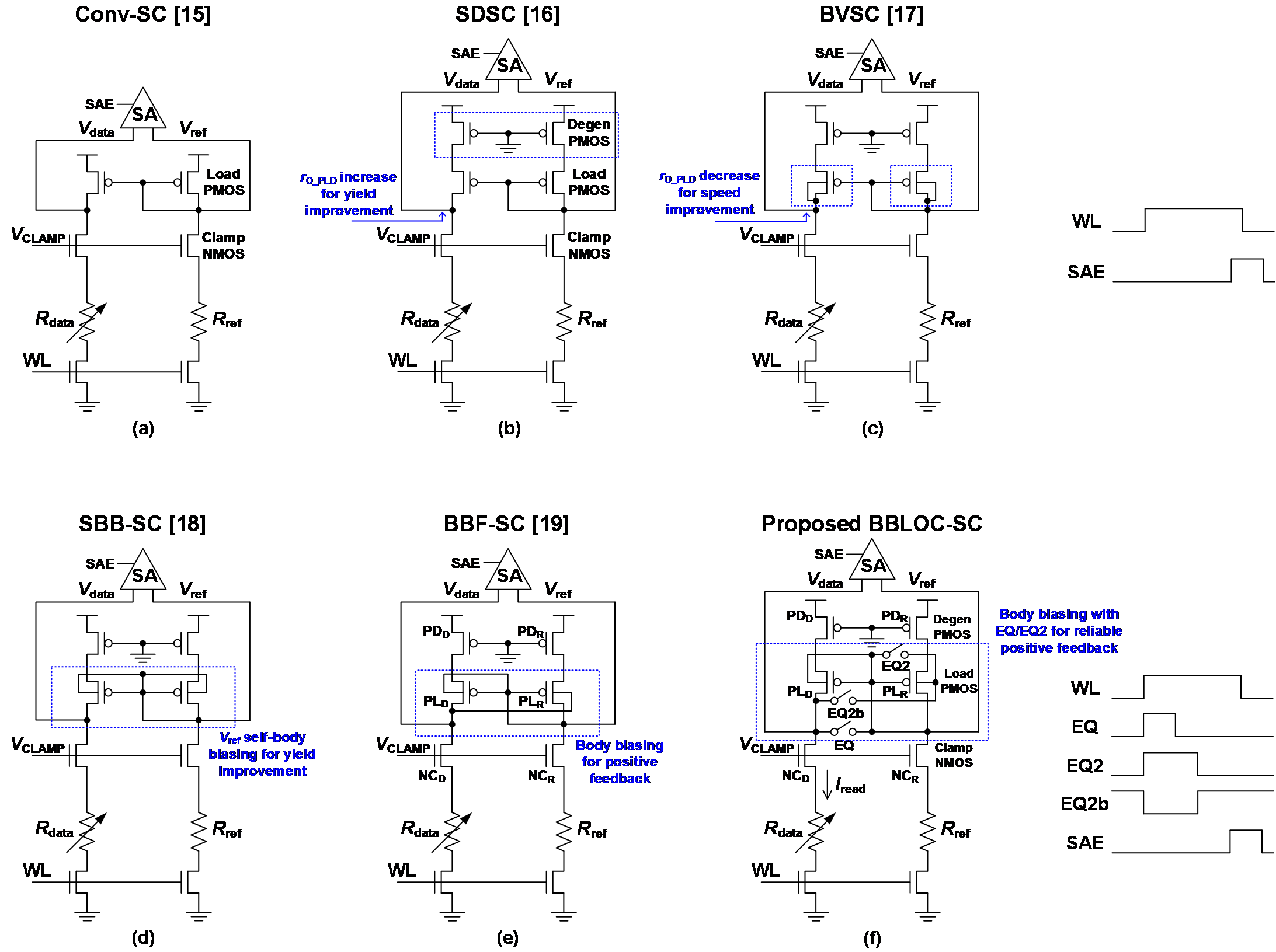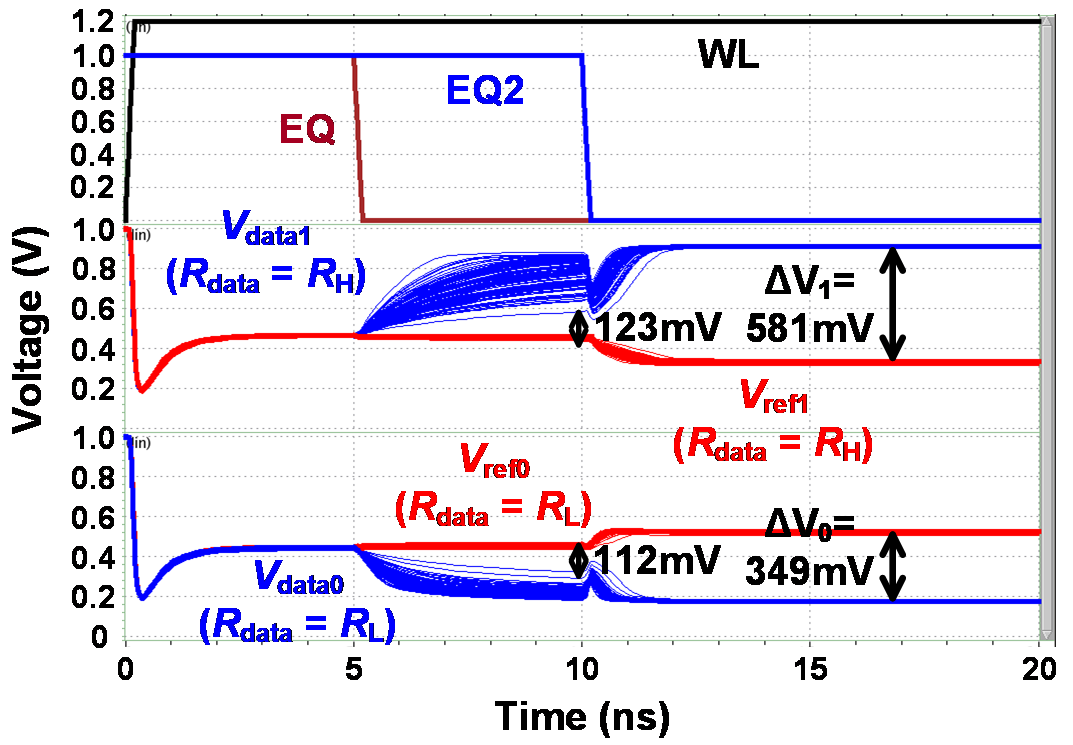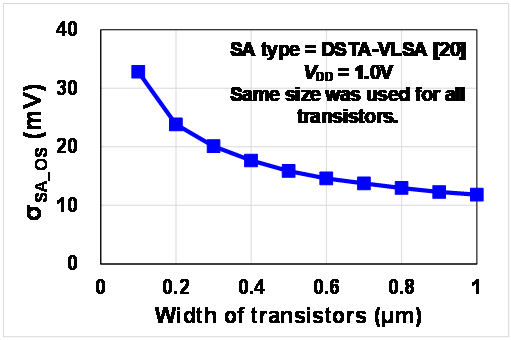Mobile QR Code1. (Department of EE, Incheon National University, Incheon 22012, Korea)

Body-biasing, latch offset cancellation, positive feedback, sensing circuit, resistive device, spin-transfer-torque magnetoresistive random access memory (STT-MRAM)

## I. INTRODUCTION

In order to address the leakage power consumption problem of the conventional static random access memory (RAM) and dynamic RAM, various non-volatile memories have been emerged, such as spin-transfer-torque magnetoresistive RAM (STT-MRAM), resistive RAM, and phase-change RAM. Among them, STT-MRAM is considered as a leading candidate for on-chip memory applications because of its intrinsic characteristics of non-volatility, high endurance, high speed, high density, long retention time, great CMOS compatibility, and no need to use a charge pump (meaning logic voltage is sufficient for write operation) (1-9). As shown in Fig. 1, STT-MRAM bit-cell is composed of one transistor one magnetic tunnel junction (MTJ), and the resistance of MTJ can be low resistance (R$_{\mathrm{L}}$) or high resistance (R$_{\mathrm{H}}$) according to the magnetization direction of the free layer compared to that of the pinned layer. However, designing a sensing circuit (SC) that achieves sufficient read yield is challenging because of the increased process variation, decreased read current (I$_{\mathrm{read}}$), and small tunnel magnetoresistance (TMR) ratio, where the read yield in this paper means the read access pass yield in sigma for a single cell, and the TMR is defined as (R$_{\mathrm{H}}$ - R$_{\mathrm{L}}$)/R$_{\mathrm{L}}$ ${\times}$ 100. To date, the reported MTJ R$_{\mathrm{L}}$ value, resistance variability (1σ, standard deviation), and TMR in the literature are in the ranges of 2-6 kΩ, 4-8%, and 80-200%, respectively (6,7, 10-14).

Fig. 2(a) shows a conventional SC (Conv-SC) consisting of clamp NMOS and current-mirror-type load PMOS (15). To overcome the deteriorated read yield caused by the process variation and short channel effect, Kim {et al.} (16) proposed the source degeneration SC (SDSC) (Fig. 2(b)) to increase the output resistance (R$_{\mathrm{O\_PLD}}$) of load PMOS because the SC output voltage difference (ΔV) between data voltage (V$_{\mathrm{data}}$) and reference voltage (V$_{\mathrm{ref}}$) is proportional to R$_{\mathrm{O\_PLD}}$. Ren {et al.} (17) proposed the body-voltage SC (BVSC) (Fig. 2(c)) to improve the sensing speed by sacrificing R$_{\mathrm{O\_PLD}}$, thereby degrading the read yield. Kim {et al.} (18) proposed the self-body biasing SC (SBB-SC) (Fig. 2(d)) to adaptively optimize V$_{\mathrm{ref}}$ without additional body voltage generator, thereby improving the read yield. Yang {et al.} (19) proposed the body-biasing feedback SC (BBF-SC) (Fig. 2(e)) to improve ΔV by using positive feedback. Even though the concept is good, the read yield is significantly degraded because the positive feedback operation begins at the initial sensing period meaning that ΔV is not stabilized.

Fig. 1. One transistor one magnetic tunnel junction (MTJ) STT-MRAM bit-cell having two states. This bit-cell represents both in-plane and perpendicular MTJs.Fig. 2. Simplified circuit diagrams of the previous sensing circuits (SCs). (15-19) and proposed SC for STT-MRAM (a) Conventional SC (Conv-SC) (15), (b) Source degeneration SC (SDSC) (16), (c) Body-voltage SC (BVSC) (17), (d) Self-body biasing SC (SBB-SC) (18), (e) Body-biasing feedback SC (BBF-SC) (19), (f) Proposed body-biasing-based latch offset cancellation SC (BBLOC-SC).In this paper, a novel body-biasing-based latch offset cancellation SC (BBLOC-SC) (Fig. 2(f)) that is capable of canceling the offset voltage caused by the latch sense amplifier (SA) is proposed, and compared with the previous SCs (15-19). The latch offset cancellation principle of the proposed BBLOC-SC is that V$_{\mathrm{data}}$ and V$_{\mathrm{ref}}$ are amplified to almost rail-to-rail voltages by the positive feedback with zero SC offset voltage when second equalization (EQ2) signal is deactivated, thereby the latch offset voltage due to SA does not affect the read yield. This advantage not only improves the read yield but also allows the minimum sized transistors to be used in SA designs, saving area and power.

The remainder of this paper is organized as follows. Section II describes the proposed BBLOC-SC. Section III presents the simulation results and comparison. Finally, Section IV concludes the paper.

Fig. 3. Transient response of the proposed BBLOC-SC. For this simulation, 28-nm model parameters, V$_{\mathrm{DD}}$ of 1.0 V, V$_{\mathrm{CLAMP}}$ of 0.6 V (I$_{\mathrm{read}}$ = 21.2 ${\mu}$A at state 1 (R$_{\mathrm{data}}$ = R$_{\mathrm{H}}$) and 25 $^{\circ}$C), boosted word line (WL) voltage of 1.2 V, R$_{\mathrm{L}}$ of 3 kΩ, R$_{\mathrm{H}}$ of 6 kΩ (TMR = 100%), 4% MTJ resistance variation, cell per bit line (BL) of 1024, width/length (W/L) of degeneration PMOS of 0.5 ${\mu}$m/0.1 ${\mu}$m, W/L of load PMOS of 4.0 ${\mu}$m/0.1 ${\mu}$m, W/L of clamp NMOS of 4.0 ${\mu}$m/0.1 ${\mu}$m, and 100 sets of Monte Carlo HSPICE simulations were used.## II. Proposed BBLOC-SC

Fig. 2(f) and Fig. 3 show the simplified circuit diagram and transient response of the proposed BBLOC-SC, respectively. When an STT-MRAM bit-cell is selected, a word line (WL) is activated. At the same time, the EQ and EQ2 signals are activated. The EQ activation at the initial sensing period is intended not only to improve the sensing speed (preventing V$_{\mathrm{data}}$ drop to GND due to capacitance mismatch between V$_{\mathrm{data}}$ and V$_{\mathrm{ref}}$ nodes) (15) but also to stabilize ΔV before the positive feedback begins. If the positive feedback is initiated when ΔV is negative, the sensing operation fails. Thus, the positive feedback starting point needs to be controlled. While the EQ signal is high, V$_{\mathrm{data}}$ and V$_{\mathrm{ref}}$ are connected together. Thus, the positive feedback is not started and these two voltages are stabilized (charging all nodes in the signal path properly). If the EQ2 signal is assumed not to be activated, after the EQ signal is deactivated, V$_{\mathrm{data0}}$ (V$_{\mathrm{data1}}$) starts to decrease (increase) and V$_{\mathrm{ref0}}$ (V$_{\mathrm{ref1}}$) starts to increase (decrease), where V$_{\mathrm{data0}}$ and V$_{\mathrm{ref0}}$ are for state 0 (R$_{\mathrm{data}}$ = R$_{\mathrm{L}}$) and V$_{\mathrm{data1}}$ and V$_{\mathrm{ref1}}$ are for state 1 (R$_{\mathrm{data}}$ = R$_{\mathrm{H}}$). Then, because the body of PL$_{\mathrm{D}}$ (PL$_{R}$) is connected to V$_{\mathrm{ref}}$ (V$_{\mathrm{data}}$), the positive feedback occurs, leading to ΔV amplification to be much higher than the offset voltage of the latch SA (σ$_{\mathrm{SA\_OS}}$). More details about the positive feedback operation can be found in the reference texts (see (19)). However, without EQ2 scheme, when ΔV is very small, the positive feedback can be started, which can lead to sensing failure. To further improve the read yield, the EQ2 scheme is employed in the BBLOC-SC. After the EQ signal is deactivated but still the EQ2 signal is being activated, ΔV is amplified without the positive feedback because both bodies of PL$_{\mathrm{D}}$ and PL$_{R}$ are connected to V$_{\mathrm{ref}}$. This structure is the same as the SBB-SC (18). In addition, when the EQ2 signal is deactivated, the charge injection and clock feedthrough occur and it further improves the read yield by balancing state 0 and state 1, which will be described later.

Fig. 3 shows that ΔV is amplified to 112 mV and 123~mV at 10 ns when R$_{\mathrm{data}}$ is R$_{\mathrm{L}}$ and R$_{\mathrm{H}}$, respectively. After the EQ2 is deactivated, the amplified ΔV (ΔV$_{0}$ = 112 mV and ΔV$_{1}$ = 123 mV) is further amplified to a much higher voltage (ΔV$_{0}$ = 349 mV and ΔV$_{1}$ = 581 mV) than the typical latch SA offset voltage of 20 mV (1σ = σ$_{\mathrm{SA\_OS}}$ = 20 mV) (20) by the positive feedback, because the body of PL$_{\mathrm{D}}$ (PL$_{R}$) is connected to V$_{\mathrm{ref}}$ (V$_{\mathrm{data}}$). Note that when the small ΔV is further amplified by the positive feedback, zero SC offset voltage is achieved because the same pairs of degeneration PMOS (PD$_{\mathrm{D}}$, PD$_{R}$), load PMOS (PL$_{\mathrm{D}}$, PL$_{R}$) and clamp NMOS (NC$_{\mathrm{D}}$, NC$_{R}$) are used before and after the EQ2 signal is deactivated. In other words, because the BBLOC-SC amplifies ΔV to almost rail-to-rail voltages by positive feedback with zero SC offset voltage and the amplified ΔV is much higher than the latch offset voltage caused by SA, the latch offset cancellation is achieved.

Table 1. Read yield and power consumption comparison according to SC and TMR when V$_{\mathrm{CLAMP}}$ = 0.6 V (I$_{\mathrm{read}}$ = 21.2 ${\mu}$A at state 1 (R$_{\mathrm{data}}$ = R$_{\mathrm{H}}$) and 25 $^{\circ}$C) and σ$_{\mathrm{SA\_OS}}$ = 20 mV.

 Read yield (σ) (Avg. power (μW)) TMR (%) 60 80 100 120 Conv-SC (15) 1.073σ (52.74) 1.358σ (51.28) 1.618σ (49.91) 1.838σ (48.62) SDSC (16) 1.917σ (50.79) 2.334σ (49.40) 2.770σ (48.10) 3.108σ (46.88) BVSC (17) 1.328σ (53.13) 1.626σ (51.83) 1.887σ (50.64) 2.103σ (49.54) SBB-SC (18) 1.985σ (52.74) 2.417σ (51.24) 2.710σ (49.83) 3.196σ (48.52) BBF-SC (19) 0.000σ (51.20) 0.000σ (49.85) 0.060σ (48.62) 0.111σ (47.49) BBLOC-SC w/o SD & w/ EQ2 1.216σ (53.57) 1.493σ (52.21) 1.748σ (50.95) 1.994σ (49.78) BBLOC-SC w/ SD & w/o EQ2 1.948σ (51.26) 2.378σ (49.92) 2.820σ (48.70) 3.239σ (47.57) BBLOC-SC (w/ SD & w/ EQ2) 2.032σ (51.70) 2.569σ (50.31) 3.062σ (49.04) 3.353σ (47.87)

Table 2. Read yield and power consumption comparison according to SC and TMR when V$_{\mathrm{CLAMP}}$ = 0.5 V (I$_{\mathrm{read}}$ = 14.0~${\mu}$A at state 1 (R$_{\mathrm{data}}$ = R$_{\mathrm{H}}$) and 25 $^{\circ}$C) and σ$_{\mathrm{SA\_OS}}$ = 20 mV).

 Read yield (σ) (Avg. power (μW)) TMR (%) 60 80 100 120 Conv-SC (15) 0.832σ (34.18) 1.051σ (33.35) 1.248σ (32.56) 1.428σ (31.82) SDSC (16) 1.352σ (33.58) 1.675σ (32.72) 1.993σ (31.91) 2.239σ (31.15) BVSC (17) 0.874σ (34.63) 1.082σ (33.84) 1.256σ (33.11) 1.411σ (32.44) SBB-SC (18) 1.419σ (34.50) 1.765σ (33.62) 2.054σ (32.79) 2.246σ (32.01) BBF-SC (19) 0.000σ (33.93) 0.000σ (33.18) 0.000σ (32.48) 0.000σ (31.84) BBLOC-SC w/o SD & w/ EQ2 0.917σ (34.87) 1.190σ (34.04) 1.395σ (33.28) 1.591σ (32.58) BBLOC-SC w/ SD & w/o EQ2 1.451σ (34.32) 1.706σ (33.52) 1.986σ (32.77) 2.290σ (32.09) BBLOC-SC (w/ SD & w/ EQ2) 1.482σ (34.31) 1.825σ (33.49) 2.142σ (32.74) 2.576σ (32.03)

## III. Simulation Results and Comparison

HSPICE Monte Carlo simulations were performed using the industry-compatible 28-nm model parameters. A nominal supply voltage (V$_{\mathrm{DD}}$) of 1.0 V and a boosting WL voltage of 1.2 V were used. The simulations were performed at ${-}$45 $^{\circ}$C and 90 $^{\circ}$C so that the result of read yield includes all temperature variation effects as well. To consider the parasitic resistance and capacitance in bit line (BL), 1024 cells per BL were simulated with parasitic resistance and capacitance components. The read yield in this paper represents the minimum value between read yield at state 0 & ${-}$45 $^{\circ}$C, at state 0 & 90 $^{\circ}$C, at state 1 & ${-}$45 $^{\circ}$C, and at state 1 & 90 $^{\circ}$C. R$_{\mathrm{L}}$ of 3 kΩ and R$_{\mathrm{H}}$ of 6 kΩ (corresponding TMR of 100%) were used for default MTJ model (14). For different TMR simulation, R$_{\mathrm{H}}$ value was adjusted. To consider the MTJ resistance variation, a standard deviation of 4% was used (12). For the size of transistors, width/length (W/L) of degeneration PMOS of 0.5 ${\mu}$m/0.1 ${\mu}$m (which is the optimal size for maximizing the read yield), W/L of load PMOS of 4.0 ${\mu}$m/0.1 ${\mu}$m, W/L of clamp NMOS of 4.0~${\mu}$m/0.1 ${\mu}$m, W/L of BL and source line (SL) switches of 2.0 ${\mu}$m/0.03 ${\mu}$m, and W/L of EQ and EQ2 transmission gate switches of 2.0 ${\mu}$m/0.03 ${\mu}$m were used.

Table 1 and 2 show the read yield and power consumption comparison according to SC and TMR when the clamp voltage (V$_{\mathrm{CLAMP}}$) for the gate of clamp NMOS is 0.6 V and 0.5 V, respectively. Power consumption is an average power consumption for 20 ns. I$_{\mathrm{read}}$ can be controlled by V$_{\mathrm{CLAMP}}$ because the previous SCs and proposed BBLOC-SC use the current-mode (constant-voltage) sensing (6). When V$_{\mathrm{CLAMP}}$ is 0.6 V and 0.5 V, I$_{\mathrm{read}}$ flowing through R$_{\mathrm{H}}$ MTJ at 25 $^{\circ}$C becomes 21.2 ${\mu}$A and 14.0 ${\mu}$A, respectively. First, it can be seen that the employment of the SD scheme improves the read yield by comparing between the Conv-SC and the SDSC and between the BBLOC-SC without SD and with EQ2 schemes (w/o SD & w/ EQ2) and BBLOC-SC w/ SD & w/ EQ2. Second, it can be seen that using the EQ scheme in the BBLOC-SC improves the read yield significantly by comparing the previous SCs (15-19) and the BBLOC-SC w/ SD & w/o EQ2. It is worth noting here that some read yield of the BBLOC-SC w/ SD & w/o EQ2 is slightly lower than that of the SBB-SC. It is because of the stability issue described earlier and it is overcome by employing the EQ2 scheme. Finally, it can be seen that the EQ2 scheme in the BBLOC-SC further improves the read yield by comparing the BBLOC-SC w/ SD & w/o EQ2 and the BBLOC-SC w/ SD & w/ EQ2. Thus, the Table 1 and 2 clearly prove that the proposed BBLOC-SC (w/ SD & w/ EQ2) has the highest read yield without using higher power, regardless of TMR and I$_{\mathrm{read}}$.

Table 3. ΔV$_{0}$ and ΔV$_{1}$ of the SDSC, BVSC, and proposed BBLOC-SC according to V$_{\mathrm{th}}$ mismatch of load PMOS when TMR = 100%, V$_{\mathrm{CLAMP}}$ = 0.6 V, and 25 $^{\circ}$C.

 Single corner simulation (only load PMOS Vth mismatch is applied) Vth mismatch of load PMOS (mV) 0 18 20 24 25 26 27 SDSC (16) ΔV0 (mV) 119 74.8 67.2 48.3 42.5 36.1 28.9 ΔV1 (mV) 374 63.1 30.5 -19.5 -28.7 -36.9 -43.9 SBB-SC (18) ΔV0 (mV) 244 188 176 145 135 124 112 ΔV1 (mV) 368 137 102 30.3 12.1 -6.05 -24 BBLOC-SC (w/ SD & w/ EQ2) ΔV0 (mV) 349 345 345 344 344 344 344 ΔV1 (mV) 581 579 579 579 578 578 -342

Table 4. ΔV$_{0}$ and ΔV$_{1}$ of the SDSC, BVSC, and proposed BBLOC-SC according to V$_{\mathrm{th}}$ mismatch of clamp NMOS when TMR = 100%, V$_{\mathrm{CLAMP}}$ = 0.6 V, and 25 $^{\circ}$C.

 Single corner simulation (only clamp NMOS Vth mismatch is applied) Vth mismatch of clamp NMOS (mV) 0 35 37 38 39 40 41 SDSC (16) ΔV0 (mV) 119 51.4 38.8 31.5 23.5 14.9 5.53 ΔV1 (mV) 374 48.5 34.6 28.3 22.5 17.1 12.2 SBB-SC (18) ΔV0 (mV) 244 83.5 57.4 44.1 30.6 17 3.32 ΔV1 (mV) 368 68.5 46.9 36.2 25.4 14.7 4.1 BBLOC-SC (w/ SD & w/ EQ2) ΔV0 (mV) 349 346 346 346 346 345 -561 ΔV1 (mV) 581 566 565 564 564 563 563

Table 5. Endurable V$_{\mathrm{th}}$ mismatch of the BBLOC-SC for correct sensing operation according to NMOS width : PMOS width of EQ2 switch.

 Single corner simulation NMOS width : PMOS width of EQ2 switch (μm) 0.5:3.5 1.0:3.0 2.0:2.0* 3.0:1.0 3.5:0.5 BBLOC-SC (w/ SD & w/ EQ2) Endurable load PMOS Vth mismatch (mV) (worst state) 23 (state 1) 24 (state 1) 26 (state 1) 28 (state 1) 29 (state 1) Endurable clamp NMOS Vth mismatch (mV) (worst state) 37 (state 1) 38 (state 1) 40 (state 0) 37 (state 0) 36 (state 0)

* Default size used in this paper.

There are two reasons for the improved read yield by the EQ2 scheme. One is because the stability issue is eliminated (in this case, the read yield of the BBLOC-SC is the same as that of the SBB-SC if σ$_{\mathrm{SA\_OS}}$ is not considered), and the other is because the charge injection and clock feedthrough of the EQ2 switch operation balance state 0 and state 1, thereby improving the read yield further.

Table 3 (Table 4) shows ΔV$_{0}$ and ΔV$_{1}$ of the SDSC, SBB-SC, and proposed BBLOC-SC according to threshold voltage (V$_{\mathrm{th}}$) mismatch of load PMOS (clamp NMOS). Considering σ$_{\mathrm{SA\_OS}}$ of 20 mV, ΔV should be at least 30 mV for correct sensing operation. In this regard, the endurable load PMOS V$_{\mathrm{th}}$ mismatch of the SDSC, SBB-SC, and BBLOC-SC is 20 mV, 24 mV, and 26 mV, respectively. In the same manner, the endurable clamp NMOS V$_{\mathrm{th}}$ mismatch of the SDSC, SBB-SC, and BBLOC-SC is 37 mV, 38 mV, and 40 mV, respectively. Thus, the Table 3 and 4 clearly show the better mismatch tolerant characteristic of the proposed BBLOC-SC compared to the previous SCs. Note that the endurable load PMOS V$_{\mathrm{th}}$ mismatch of 26 mV is smaller than the endurable clamp NMOS V$_{\mathrm{th}}$ mismatch of 40 mV in case of the BBLOC-SC. It means that the read yield of the BBLOC-SC is much sensitive to the load PMOS V$_{\mathrm{th}}$ mismatch than the clamp NMOS. Also, the Table 3 shows that the worst case happens at state 1 (ΔV$_{1}$). In this respect, if the state 0 and state 1 are well balanced, the read yield can be improved. Table 5 shows that adjusting the ratio between NMOS width and PMOS width of EQ2 switch can be used for balancing because this ratio makes different charge injection and clock feedthrough effect on the V$_{\mathrm{data}}$ and V$_{\mathrm{ref}}$ nodes. If the ratio increases, the endurable load PMOS V$_{\mathrm{th}}$ mismatch increases by trading off the endurable clamp NMOS V$_{\mathrm{th}}$ mismatch. In this paper, the same size of NMOS and PMOS for EQ2 switch is selected for symmetric layout design, and because of this balancing effect, the read yield of the BBLOC-SC is higher than that of the SBB-SC.

Fig. 4. σ$_{\mathrm{SA\_OS}}$ of DSTA-VLSA according to width of transistors. For this simulation, 28-nm model parameters, V$_{\mathrm{DD}}$ of 1.0 V, SA enable signal rise time of 100 ps, V$_{\mathrm{BL}}$ = 0.5 V were used, and same size was used for all transistors (width = variable, length = 0.03 ${\mu}$m).The output voltages (V$_{\mathrm{data}}$ and V$_{\mathrm{ref}}$) of SC used for STT-MRAM are in the range from GND to V$_{\mathrm{DD}}$ (as illustrated in Fig. 3). In this case, employing the voltage-latched SA with double switches and transmission gate access transistors (DSTA-VLSA) (20) that has no sensing dead zone is a good choice. Fig. 4 shows σ$_{\mathrm{SA\_OS}}$ of DSTA-VLSA according to width of transistors. For this simulation, 28-nm model parameters, V$_{\mathrm{DD}}$ of 1.0 V, SA enable signal rise time of 100 ps, V$_{\mathrm{BL}}$ = 0.5 V were used, and same size was used for all transistors (width = variable, length = 0.03 ${\mu}$m). This figure clearly shows that σ$_{\mathrm{SA\_OS}}$ is inversely proportional to the width of transistors. For a smaller σ$_{\mathrm{SA\_OS}}$ (for a higher read yield), a larger area overhead caused by the SA is unavoidable. It also results in a higher power consumption because of the increased loading capacitances.

If σ$_{\mathrm{SA\_OS}}$ does not affect the read yield, the minimum sized transistors can be used in SA designs, thereby saving area and power. Fig. 5 shows the read yield of the BBLOC-SC, SBB-SC, and SDSC according to σ$_{\mathrm{SA\_OS}}$. Unlike the read yield of the SBB-SC and SDSC that decreases as σ$_{\mathrm{SA\_OS}}$ increases, the read yield of the proposed BBLOC-SC remains constant regardless of σ$_{\mathrm{SA\_OS}}$. Thus, the BBLOC-SC can improve not only the read yield but also the area and power efficiency.

## IV. CONCLUSIONS

This paper proposes a novel BBLOC-SC that has the major advantage of latch SA offset cancellation by amplifying the SC output voltages (V$_{\mathrm{data}}$ and V$_{\mathrm{ref}}$) to almost rail-to-rail voltages with zero SC offset voltage, thereby making the proposed BBLOC-SC to be tolerant to the offset voltage caused by latch SA. The simulation results prove that the BBLOC-SC can achieve a much higher read yield compared to the previous SCs without using higher power, regardless of TMR and I$_{\mathrm{read}}$. For example, when TMR is 120% and I$_{\mathrm{read}}$ is 14 ${\mu}$A, the read yields of 2.239σ (SDSC), 2.246σ (SBB-SC), and 2.576σ (BBLOC-SC) correspond to sensing error rates of 1.26%, 1.24%, and 0.50%, respectively. It means that the BBLOC-SC produces 2.52x and 2.48x improvement in the read yield compared to the SDSC and SBB-SC, respectively. The only drawback of the BBLOC-SC is the increased area caused by the inclusion of EQ2 switches, and its area overhead is estimated to 13% from the SC viewpoint. Hence, the proposed BBLOC-SC can be applied for deep submicrometer STT-MRAM applications.

### ACKNOWLEDGMENTS

This work was supported by the National Research Foundation of Korea (NRF) grant funded by the Korea government (MSIT) (No. 2020R1F1A1060395). The EDA Tool was supported by the IC Design Education Center.

### REFERENCES

1
Hosomi M., 2005, A novel nonvolatile memory with spin torque transfer magnetization switching: Spin-RAM, In Proc. IEEE Int. Electron Devices Meeting (IEDM) Tech. Dig., Vol. , No. , pp. 459-4622
Lin C. J., 2009, 45 nm low power CMOS logic compatible embedded STT MRAM utilizing a reverse-connection 1T/1MTJ cell, in IEEE Int. Electron Devices Meeting (IEDM) Tech. Dig., pp. 279-2823
Tsuchida K., 2010, A 64Mb MRAM with clamped-reference and adequate-reference schemes, in IEEE Int. Solid-State Circuits Conf. (ISSCC) Dig. Tech. Papers, pp. 258-2594
Ikeda S., Jul 2010, A perpendicular-anisotropy CoFeB-MgO magnetic tunnel junction, Nature Materials, pp. 721-7245
Kang S. H., Lee K., 2013, Emerging materials and devices in spintronic integrated circuits for energy-smart mobile computing and connectivity, Acta Materialia, Vol. 61, No. 3, pp. 952-9736
Na T., Jan 2021, STT-MRAM sensing: a review, IEEE Trans. Circuits Syst. II Exp. Briefs, Vol. 68, No. 1, pp. 12-187
Kang S. H., Park C., 2017, MRAM: enabling a sustainable device for pervasive system architectures and applications, in IEEE Int. Electron Devices Meeting (IEDM) Tech. Dig., pp. 38.2.1-38.2.48
Wei L., 2019, A 7Mb STT-MRAM in 22FFL FinFET technology with 4ns read sensing time at 0.9V using write-verify-write scheme and offset-cancellation sensing technique, in IEEE Int. Solid-State Circuits Conf. (ISSCC) Dig. Tech. Papers, pp. 214-2159
Chih Y.-D., 2020, A 22nm 32Mb embedded STT-MRAM with 10ns read speed, 1M cycle write endurance, 10 years retention at 150$^\circ$C and high immunity to magnetic field interference, in IEEE Int. Solid-State Circuits Conf. (ISSCC) Dig. Tech. Papers, pp. 222-22410
Lu Y., 2015, Fully functional perpendicular STT-MRAM macro embedded in 40 nm logic for energy-efficient IOT applications, In Proc. IEEE Int. Electron Devices Meeting (IEDM) Tech. Dig., pp. 26.1.1-26.1.411
Kim C., 2015, A covalent-bonded cross-coupled current-mode sense amplifier for STT-MRAM with 1T1MTJ common source-line structure array, in IEEE Int. Solid-State Circuits Conf. (ISSCC) Dig. Tech. Papers, pp. 1-312
Rizzo N., 2010, Toggle and spin torque: MRAM at Everspin technologies, Non-volatile Memories Workshop13
Rizzo N. D., Jul 2013, A fully functional 64 Mb DDR3 ST-MRAM built on 90 nm CMOS technology, IEEE Trans. Magn., Vol. 49, No. 7, pp. 4441-444614
Lee K., Kang S. H., Jan 2011, Development of embedded STT-MRAM for mobile system-on-chips, IEEE Trans. Magn., Vol. 47, No. 1, pp. 131-13615
Kim J. P., 201, A 45nm 1Mb embedded STT-MRAM with design techniques to minimize read-disturbance, in IEEE Symp. VLSI Circuits Dig. Tech. Papers1, pp. 296-29716
Kim J., Jan 2012, A novel sensing circuit for deep submicron spin transfer torque MRAM (STT-MRAM), IEEE Trans. Very Large Scale Integr. (VLSI) Syst., Vol. 20, No. 1, pp. 181-18617
Ren F., 2012, A body-voltage-sensing-based short pulse reading circuit for spin-torque transfer RAMs (STT-RAMs), in Int. Symp. Quality Electron Design (ISQED), pp. 275-28218
Kim J., Jul 2014, STT-MRAM sensing circuit with self-body biasing in deep submicron technologies, IEEE Trans. Very Large Scale Integr. (VLSI) Syst., Vol. 22, No. 7, pp. 1630-163419
Yang L., May 2015, A body-biasing of readout circuit for STT-RAM with improved thermal reliability, in Proc. IEEE Int. Symp. Circuits Syst. (ISCAS), pp. 1530-153320
Na T., Woo S.-H., Kim J., Jeong H., Jung S.-O., Feb 2014, Comparative study of various latch-type sense amplifiers, IEEE Trans. Very Large Scale Integr. (VLSI) Syst., Vol. 22, No. 2, pp. 425-429## Author

received the B.S. and Ph.D. degrees in Electrical & Electronic Engineering from Yonsei University, Seoul, Republic of Korea, in 2012 and 2017, respectively.

From 2017 to 2019, he was with Samsung Electronics Co., Ltd., Hwasung, Republic of Korea, where he worked on phase-change random access memory (PRAM) and high-performance NAND (ZNAND) core circuit designs.

Since 2019, he has been a professor at Incheon National University, Incheon, Republic of Korea.

His current research interests are focused on process-voltage-temperature variation tolerant and low-power circuit designs for memory, microcontroller unit, and neuromorphic SoC.